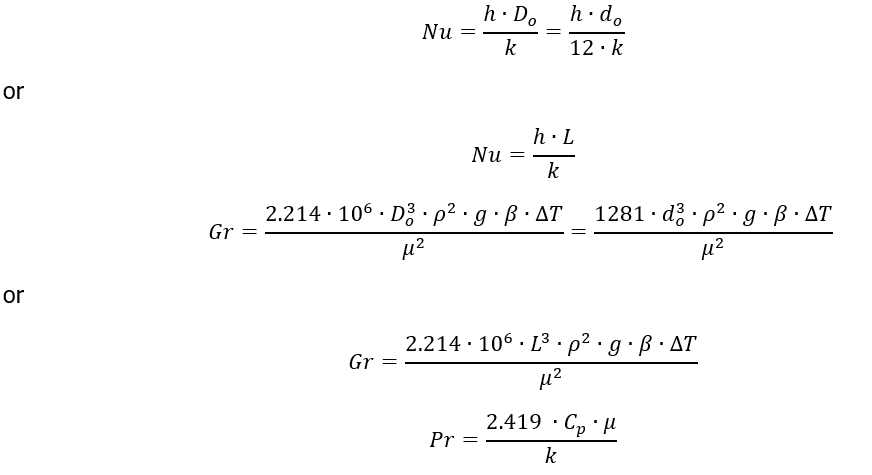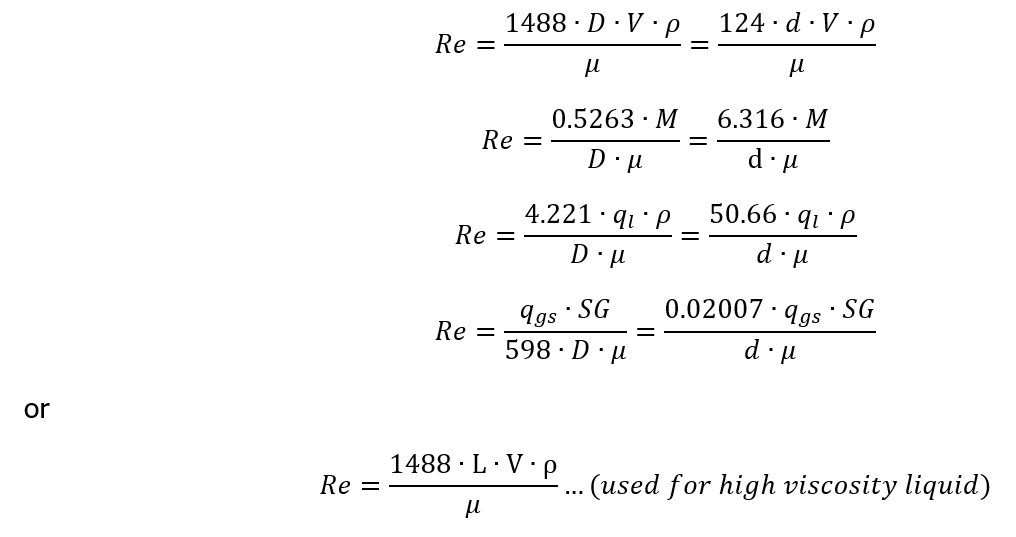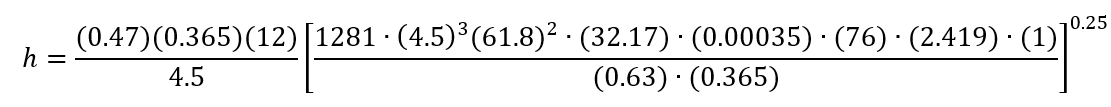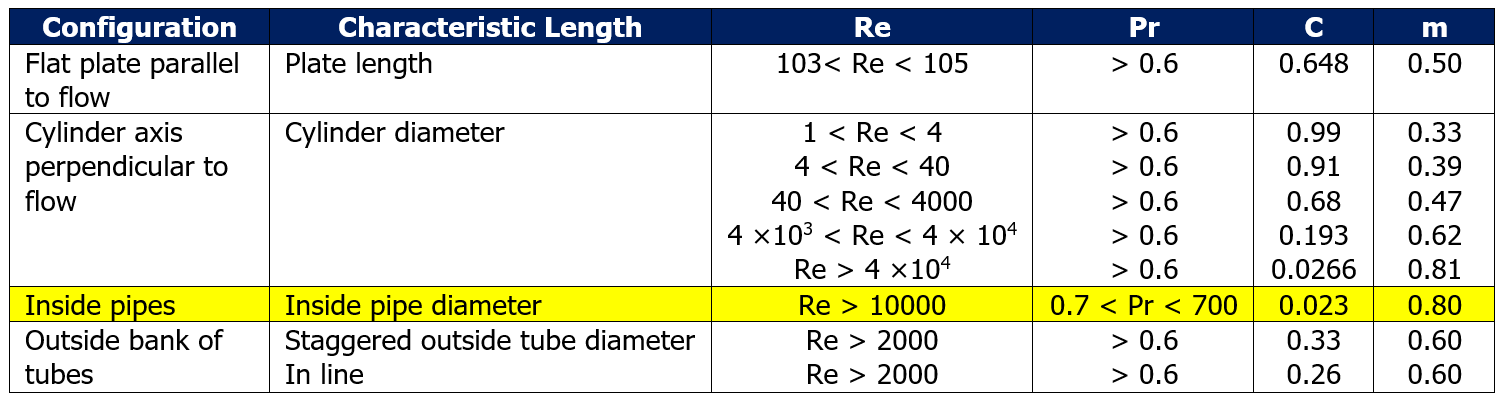# What is Convection and Its Problem Example

In previous post we learned what is conduction and its example on heat loss through a pipe wall with insulation. In this post, I want to share what is convection and its problem example.

Convection is heat transfer between a solid and an adjacent fluids develops as a result of fluid molecular movement. Cold molecules replace hot molecules as they leave the solid surface. A thin layer or film next to the solid surface is where most of the resistance to this type of heat transfer occurs. Even though the bulk fluid flow is extremely turbulent, this layer still exists.

Convective heat transport is governed by Newton’s law of cooling.

Q = h ∙A∙ ∆T

Where:

Q = heat transfer (Btu/hr)

h = heat transfer coefficient [Btu/(hr∙ ft2oF)]

A = area (ft2)

∆T = temperature difference (oF)

Convective heat transfer is divided into two types, natural or free convection and forced convection.

## Natural or Free Convection

Natural or free convection happens when the only force promoting the fluid flow results from temperature differences in the fluid. The heat transfer coefficient is obtained from the Nusselt equation under this condition.

Nu = C (Gr ∙ Pr)m

Where:Where:

Nu        = Nusselt number (dimensionless)

Gr         = Grashof number (dimensionless)

Pr         = Prandtl number (dimensionless)

C          = constant

m         = constant

h          = heat transfer coefficient [Btu/(hr∙ ft2oF)]

Do              = outside diameter of pipe or cylinder (ft)

do         = outside diameter of pipe or cylinder or fin (in)

k          = thermal conductivity (Btu/[hr∙ft2∙F/ft])

L          = length of heat transfer surface (ft)

ρ          = density of fluid (lb/ft3)

β          = volumetric coefficient of thermal expansion (1/oF)

g          = gravity acceleration (32.17 ft/s2)

μ          = viscosity of fluid (cP)

∆T        = temperature difference (oF)

C and m constants depend on the shape and size of solid surface, the orientation of the surface to the fluid, whether the solid is hotter than the fluid or vice versa, and the magnitude of (Gr∙Pr).

The value of C and m for common condition is shown below.

## Forced Convection

Forced convection occurs when the flow of fluid adjacent to a solid is promoted by external force, such as pumping, agitation, and so on. The result is significant increase in heat transfer rate.

This correlation applies to forced convection:

Nu = C ∙Rem ∙Pr0.33

By utilize the viscosity correlation:

Nu = C ∙Rem ∙Pr0.33∙ (μb/ μw)0.14

Where:Where:

Re        = Reynolds number

D          = diameter of pipe or cylinder (ft)

V          = velocity (ft/s)

M         = mass flow rate (lb/hr)

ql            = liquid flow rate (gpm)

ggs        = gas flow rate at standard condition at 14.7 psia and 60oF (scf/day)

SG        = specific gravity

The constant C and m depend on the configuration and the type of fluid flow (laminar, intermediate, or turbulent), which is characterized by the value of Reynolds number. Value of C and m for common condition is shown below.

### Example 1 (Related to Natural or Free Convection)

What is the heat transfer coefficient for natural convection around a 4 in schedule 40 pipe surrounded by water at 190oF? Assume outside temperature (To) for the pipe is 114oF.

Solution

 Determine film temperature which is average of fluid temperature and outside solid temperature.

Tfilm = (190 + 114) / 2 = 152oF

 Determine C and m.

Outside diameter is 4.5 in or 0.375 ft. Based on table 1, C is 0.47 and m is 0.25Nu = C (Gr ∙ Pr)m

Nu = 0.47 (Gr ∙ Pr)0.25The properties of water are based on film temperature. The properties include ρ, μ, β, and k.

do = 4.5 in

ρ = 61.8 lb/ft3

g = 32.17 ft/s2

β = 0.00035 oF-1

∆T = 190 – 114 = 76oF

Cp = 1 Btu/(lb∙F)

μ = 0.63 cP

k = 0.365 Btu/[(hr∙ft2oF)/ft]

Substituting:h = 115 Btu/(hr∙ft2oF)

### Example 2 (Related to Forced Convection)

Estimate the heat transfer coefficient for 5 MMscfd natural gas with specific gravity of 0.6 flowing at 2000 psia in a 3 in schedule 80 pipe when the pipe wall temperature is 104oF and gas temperature is 72oF.

Solution

 Determine film temperature which is average of fluid temperature and pipe wall temperature.

Tfilm = (104 + 72) / 2 = 88oF

 Determine C and m.

Based on table 2, the value of C and m for configuration inside pipes is 0.023 and 0.80, respectively.Use equation for forced convection to get h

Nu = C ∙Rem ∙Pr0.33

Nu = 0.023 ∙Re0.80 ∙Pr0.33

For configuration inside pipes, we used inside pipe diameter as length.Where:

di          =  2.9 in

μ          = 0.019 cP

k          = 0.03 Btu/[hr∙ft2oF/ft]

Cp        = 0.73 Btu/(lb∙oF)

qgs        = 5 ∙ 106 scfd

Substituting:h = 200.67 Btu/(hr∙ft2oF)

That’s all this post about what is convection and its problem example. I hope you find it useful.